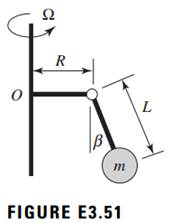### Create an Account

Home / Questions / For small oscillations determine the equation of motion and the natural frequency of the r...

# For small oscillations determine the equation of motion and the natural frequency of the rotating pendulum shown in Figure E351 that oscillates about the equilibrium position β when the angular

For small oscillations, determine the equation of motion and the natural frequency of the rotating pendulum shown in Figure E3.51 that oscillates about the equilibrium position β when the angular rotation is Ω.May 16 2020 View more View LessSubscribe To Get Solution